# 5G NR Transport Block Size (TBS) Calculation

Transport Block  in 5G NR, is nothing but the payload which is passed between the MAC and Phy Layers, specifically for the shared data channel such as PDSCH and PUSCH. A Transport Block undergoes Phy layer processing at the transmitter before being mapped onto the PDSCH for transmission over the air interface. A Transport block is added with CRC and segmented into code blcoks as shown as below. The transport The transport block consists of up to million bits and the code block consists of up to 8448 bits.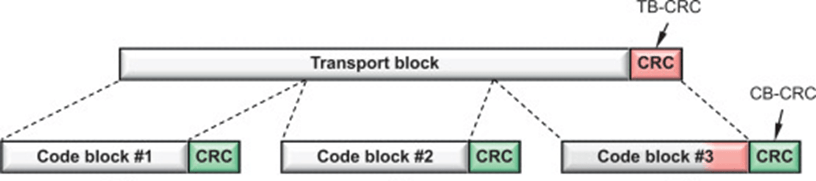Factors Affecting Transport Block Size:

Device receiving data on the PDSCH has to determine the Transport Block Size (TBS) before attempting to decode the data. The UE uses information provided by RRC signalling and Downlink Control Information (DCI) over the PDCCH depending on scheduling type i.e. semi-static or dynamic scheduling.

Following picture depicts a simplified diagram to get Transport Block Size and provided lists of parameters which affects the TB size.• No. of Layers (v)
• Modulation Order (Qm)
• Coding Rate (R)
• No. of Physical Resource Block (PRB)
• Transmission Duration

How to Calculated Transport Block Size

In 4G LTE, the transport block size is provided in a table which was a function of the MCS field and the Resource Block Allocation (PRBs). However, 5G NR support significantly larger bandwidths, together with a wide range of transmission duration and variations in the overhead depending on other features configured such as CSI-RS. It would result in a large number of tables required to handle the large dynamic range in terms of transport block sizes.

Such a scheme may also require modifications whenever some of these parameters change. Therefore, 5G NR has opted for a formula-based approach combined with a table for the smallest transport block sizes instead to obtain the necessary flexibility.Step to Calculated TBS

• The UE determines the no. of REswhich are available for data transfer within the bandwidth of a single Resource Block using following formulawhere• If the value ofis greater than 156 thenis rounded down to 156, i.e. the UE never assumes a resource allocation of more than 156 REs  within the bandwidth of a single Resource Block. The total number of REs within the bandwidth of a single RB is 12 x 14 = 168 using normal cyclic prefix.
• The finalvalue is multiplied by the number of allocated Resource Blocks to generate a value for. The number of allocated RBs is extracted from the DCI available through PDCCH, i.e. it is part of the  resource allocation.
• The value of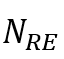represents the total no. of REs available for data transfer
• The total no. of REs available for data transfer is then converted into a no. of information bits. The no. of information bits depends upon the modulation scheme, the coding rate and the number of layers, i.e. the use of MIMO. The UE uses the following equation standardized by 3GPP .•total no. of REs available for data transfer
• R is Code Rate
• Qm is Modulation Order
• v is no. of MIMO layers
• The Modulation Order and Target Code Rate are both extracted from an MCS table
• N0. of Layers is fixed to ‘ 1’ if the PDSCH resource allocation has been received using DCI 1_0. Otherwise, the no. of Layers is obtained from the ‘DMRS Ports’ column within the “Antenna Ports’ look-up table used by DCI 1_1. The number of layers is equal to the number of allocated DMRS Ports
• If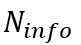<= 3824 bits, then TBS is determined based on 3GPP TS 38.214 Table and if not then TBS is determined Based on Formula. The detailed step are shown in below flow chart to determine the TBS.• https://5g-tools.com/5g-nr-tbs-transport-block-size-calculator/The threshold of 3824 bit is based upon the maximum code block size of 3840 bits which can be processed by LDPC channel coding when using ‘Base Graph 2’. CRC bits are added prior to channel coding. Transport blocks which have a size 3824 bits have a 16 bit CRC added, i.e. the total size after CRC addition can be up to 3840 bits. LDPC ‘Base Graph I’ can process a maximum code block size of 8448 bits, so transport blocks <= 3824 bits will not require segmentation prior to channel coding for both ‘Base Graph 1’ and ‘Base Graph 2’.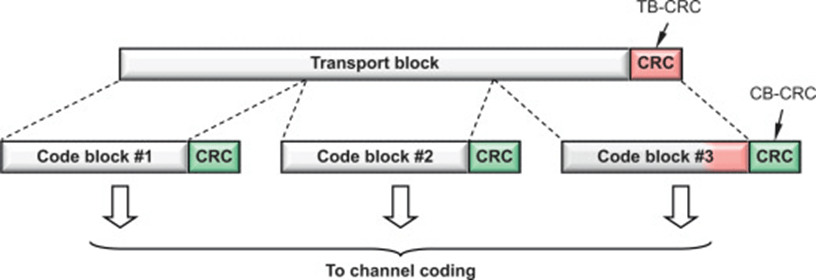• When> 3824 then a 24 bit CRC will be added rather than a 16 bit CRC. In addition, segmentation may be required prior to channel coding. When segmentation is applied, an additional 24 bit CRC is added to each segment. The following calculations account for these 24 bit CRC.is calculated as:• This equation generates a result which contains an integer number of bytes. It also generates a step size which is dependent upon the value of. A step size of 64 bits is generated for smaller values of, this increases to 128, 256, 512, … bits as the value ofincreases. The transport block size is then calculated using one of three equations:
• If Code Rate <= 0.25 then LDPC ‘Base Graph 2’ will be used (maximum code block size of 38 I 6 + 24 = 3840 bits) and the following equation is applied: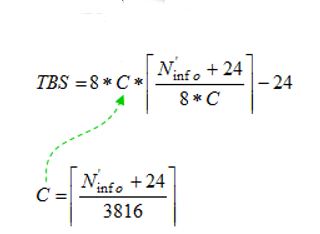• Else if> 8424 bits then LDPC ‘Base Graph I’ will be used (maximum code block size of 8424 + 24 = 8448 bits) and the following equation is applied.• Else the following equation is appliedReferences:

• 3GPP TS 38.214  NR; Physical layer procedures for data
• https://5g-tools.com/5g-nr-tbs-transport-block-size-calculator/

Related Posts: